# Your Free Math Tutor Online

Having difficulty understanding math? Looking for help in math? Or a free math tutor?

If yes, MathExpression.com is for you!

Here, we provide free math tutoring online. To do so, we have carefully created easy and understandable lessons for you. For effectively learning, we divide each lesson into four parts as listed below:Math VideosStudy Tips
Study tips that focus on important math ideas. You will save time by knowing what is necessary.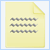Practice Questions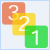Step-by-Step Solutions
Each practice question comes with step-by-step solutions with complete explanations
All these in MathExpression.com - The Free Math Tutor Online.

For each lesson, read the study tips carefully and take note of the important concepts/ideas before watching the video. These tips will guide you in your math thinking strategies.

After watching the video, try the practice questions. They are tailored to help you understand the lesson better. Have fun!

# Math Topics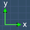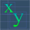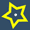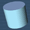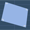# List of Math Lessons & MoreGraphs
This is an introduction to the basic parts of the coordinate plane. You'll learn about the Coordinate axis and more...
Learn how to read and write the coordinates of a point...
Learn how to draw the graph of a straight a line. This lesson will show you the basic ideas behind graphing...
Learn how to calculate the slope (gradient) of a line. You will get to familiarize with the terms 'change in x', 'change in y' and more...
This lesson shows you how the slope formula is derived and some visual examples on using it...
This lesson shows you under what circumstances a line can have negative slope, positive slope, zero or infinite slope...
This lesson shows what x and y intercepts. Also, you will see some examples on how to find the intercepts of linear equations...
In this lesson, we will examine the slope-intercept form of an equation of a line....
After learning about the slope-intercept form, let's see some examples on how to use it to draw the line of a linear equation...
This lesson shows you how to determine the equation of a line using the given information such as the slope, coordinates of a point and more....
This lesson shows you how to determine the equation of a line that is parallel to the x-axis or y-axis...Basic Algebra
This lesson introduces you to algebra. Also, you will get to know what algebra variables are all about...
This lesson shows you how to identify and combine 'like terms' in algebraic expressions...
Understand the basics behind adding 'like terms' in order to simplify algebraic expressions...
Learn how to remove brackets in algebraic expressions...
Learn how to evaluate expressions by substituting variables and perform the required calculations...
This lesson shows you the basics that you need to know when solving linear equations...
This lesson shows you some examples on how to solve linear equations to further your understanding in this topic...Exponents
This lesson shows you the basics behind the first three exponent laws. You'll learn how these laws are actually formulated...
This lesson shows you the basics behind the next three exponent laws. You'll learn how these laws are actually formulated...
This lesson shows you some examples on using the exponent laws that you have learned in the previous lessons.
This lesson shows you the basics behind fractional exponents and how they are related to roots.Symmetry
In this lesson, we will learn about line symmetry and what is line of symmetry...
This lesson shows you the ideas behind 2D Rotational Symmetry. Also, you will get to see a quick video demonstration on it...
In this lesson, we will learn about rotation symmetry for a cube and tetrahedron...Fractions
Need help in understanding fractions? This lesson will show you the basic ideas on fractions and the common terms used in describing fractions...
In this lesson, we will learn more on improper fractions and mixed fractions. Also, we will see how we can convert from improper to mixed fraction and vice versa...
This lesson is about equivalent fractions. We will learn the important ideas behind and how to find these fractions...
In this lesson, we will learn the basics behind adding fractions that have like and unlike denominators...
After understanding the basics, let's see a few more examples on how to add fractions...
In this lesson, we will learn about subtracting fractions and will be using some examples to explain how this subtraction works....
In this lesson, we will learn the basics behind multiplying fractions and will be using some examples to explain how this multiplication works...
In this lesson, we will see more examples on how to multiply fractions. Also, we will learn how to use common factor to make the multiplication easier...
In this lesson, we will use some examples to explain the basics behind dividing fractions...
In this lesson, we will see more examples on how to divide fractions. We will also see how we can use common factors to simplify the division...
This is an online fraction calculator. This calculator not only gives you the answer but also the sample solution (i.e. steps) for your reference...Volume
This lesson is an introduction to the basic ideas on how to find the volume of solids. We will be using a rectangular solid and a cylinder as examples...
In this lesson, we will learn about the volume of a cube...
In this lesson, we will learn about the volume of a cylinder...
In this lesson, we will learn about the volume of a sphere...
In this lesson, we will learn about the volume of a cone...
In this lesson, we will learn about the volume of a pyramid...
This page contains the volume formulas for a cube, rectangular solid, cylinder and sphere...Area
In this lesson, we will learn about the area of a square...
In this lesson, we will learn about the area of a rectangle...
In this lesson, we will learn about the area of a circle...
In this lesson, we will learn about the area of a parallelogram...